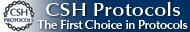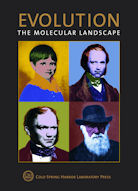BOOK COVERCLICK TO ENLARGE   Buy the BookRegister at our site www.cshlpress.com to join our Discount Program and receive 10% discounts on all website purchases.You may also be interested in:Cold Spring Harbor’s 74th Symposium
EVOLUTION
The Molecular Landscape
Edited by Bruce Stillman,
David Stewart, and
Jan Witkowski,
Cold Spring Harbor Laboratory

# Problems

Throughout most of this book, we have avoided explicit mathematics and have given mainly verbal arguments. This reflects the literature of evolutionary biology: Most papers keep any mathematics hidden in appendices or supplementary material, and most analysis of data is embedded in computer simulations and software packages. However, this superficial impression is misleading: the Evolutionary Synthesis was based on the quantitative foundation of population genetics (pp. 25–30), and now the whole range of research is based on a mathematical, statistical, and computational foundation—from study of genetically complex traits (Chapter 14) through analysis of genomic data (Chapters 15, 19, and 27) to understanding phenotypic variation (Chapters 20 and 21). To gain a full understanding of the subject, and to properly understand the primary literature, it is essential to know at least something of this quantitative basis. These problems are designed to introduce you to the range of methods that underpin evolutionary biology.

Quantitative reasoning plays several roles. First, it helps us understand and validate verbal arguments. There is a long history of plausible arguments that have proved false when described explicitly in mathematical terms. (Examples include the idea that environmental heterogeneity necessarily maintains genetic diversity [p. 508], or the reliance on group selection to explain apparently altruistic traits [p. 607]). Even simple “toy models” can give a much deeper and more accurate understanding. Second, models can show the course that evolution is likely to take. We are hardly ever able to make precise predictions, but we can estimate the approximate rate and pattern of change, and we can identify the key parameters. (For example, the load on a population depends primarily on the net rate of deleterious mutation, U; p. 552.) Finally, sophisticated statistical models are needed to make estimates from samples of genes—for example, on the geographic origin of individuals (pp. 743–749) or the location of disease genes (pp. 756–765). These problems will give you a taste of all three aspects.

Most of the problems do not require more than basic algebra. In most cases, rough answers will do; indeed, it is often best to take a simple route, making sensible approximations where possible. You will often need to make assumptions, which may not be stated in the question: Always state exactly what assumptions and approximations you are using. Often, a graphical method will help. For example, to solve the equation 0.5(qp) = pq, where q = 1 – p, just plot graphs of 0.5(q p) and pq together and see where they cross (Fig. 28.15). A few problems will need a fair amount of calculation, using a hand calculator. A spreadsheet will not be necessary and, unless you are adept at using one, could be a hindrance. The questions are designed to be solved using pencil and paper, rather than a computer.

Most of the problems do not require the more advanced material in Chapter 28: Those that do involve some basic calculus or more advanced knowledge of probability are marked *C28*. However, most of the problems do involve an understanding of the basics of probability. (What is the chance that A or B will happen? That A and B will happen? ...) So, you might find it helpful to read the outline of probability theory in Chapter 28.

Questions are graded as * (easy), ** (moderate), or *** (hard). Those marked * will be straightforward, often requiring substitution into a formula. Those marked ** will typically ask you to think through a chain of reasoning and will not provide a standard formula. Questions marked *** will require some ingenuity—and will be the more rewarding if you can solve them. HINTs are provided, which will guide you in the right direction, as well as Web Notes, which give some background. We provide detailed answers to all the problems. However, you should make every effort to solve the questions yourself before looking at these answers. (Using the hints when needed will help you get as far as possible without resorting to the answer.) Working through all these problems will be a substantial amount of work, but this is necessary if you are to really understand the foundations of the subject.

Note that cross-references to figures or tables that begin with a P (e.g., Fig. P1.2) refer to figures or tables within these Web Problems. Cross-references to figures or tables that do not begin with a P (e.g., Fig. 1.2) refer to figures or tables within the Evolution book, including the online chapters. Glossary terms are in bold.